# Artificial Neural Network based Artificial Intelligent Algorithms for Accurate Monthly Load Forecasting of Power Consumption

London Journal of Engineering Research
Volume | Issue | Compilation
Authored by Samuel Atuahene , Yukun Bao, Yao Yevenyo Ziggah, Patricia Semwaah Gyan
Classification: NA
Keywords: Energy, Load Forecasting, Back Propagation Neural Network, Radial Basis Function, Extreme Learning Machine.
Language: English

In this study, three artificial neural networks (ANN) techniques (backpropagation (BPNN), radial basis function network (RBFNN) and extreme learning machine (ELM)) were applied for accurate modeling and prediction of monthly load consumption. These models were trained for the first time on the data collected by the United State Energy Information Administration (USEIA) for five sectors from January 1973 to May 2017 (44 years). Performance evaluation of the methods was carried out using various statistical indicators including mean absolute percentage error (MAPE). The results revealed that the value of MAPE for BPNN which gave the optimum model for predicting the monthly load consumption were 0.999999885 and 0.999999069 for training and testing results respectively, ascertaining the accuracy and suitability of the model for monthly load consumption prediction.

# Artificial Neural Network based Artificial Intelligent Algorithms for Accurate Monthly Load Forecasting of Power Consumption

## ____________________________________________

### ABSTRACT

In this study, three artificial neural networks (ANN) techniques (backpropagation (BPNN), radial basis function network (RBFNN) and extreme learning machine (ELM)) were applied for accurate modeling and prediction of monthly load consumption. These models were trained for the first time on the data collected by the United State Energy Information Administration (USEIA) for five sectors from January 1973 to May 2017 (44 years). Performance evaluation of the methods was carried out using various statistical indicators including mean absolute percentage error (MAPE). The results revealed that the value of MAPE for BPNN which gave the optimum model for predicting the monthly load consumption were 0.999999885 and 0.999999069 for training and testing results respectively, ascertaining the accuracy and suitability of the model for monthly load consumption prediction.

Keywords: energy, load forecasting, back propagation neural network, radial basis function, extreme learning machine.

Author α σ: Center for Modern Information Management, School of Management Science and Engineering, Huazhong University of Science and Technology, Wuhan China.

ρ: Department of Geomatic Engineering, University of Mines and Technology, Tarkwa- Ghana.

Ѡ: Faculty of Earth Resources, China University of Geosciences, Wuhan-China.

1. ### INTRODUCTION

Energy or load demand is the most useful entity in today’s modern world. Monthly energy forecasting plays a vital role in the functioning efﬁciency of a power system, such as yearly hydro-thermal maintenance scheduling, hydro -thermal coordination, unit commitment, demand side management, security assessment, interchange evaluation and others. Occasionally, we profligate electricity whiles other times quite careful about the usage of it. Yet, the objective is to make available an uninterrupted electricity supply to users. To arrive at this objective, there is the necessity for proper evaluation of present day and future consumption of power as well as the demand.

The large number of studies have compared the forecast accuracies of alternative models based on statistical theories. As a result, a technique which can predict the demand of consumers is needed as well as the actual capacity for generating power. In line with the, scholars have applied several estimation techniques to model and predict power consumption for commercial sectors, transport sectors , as well as electrical power sectors. These techniques are used by electricity companies to predict the amount of power needed to adequately supply the demand. Techniques for predicting power consumption uses a set of known entities to produce future values for the same or other entities. These predictive models can be categorized into two main approaches which are the classical and modern methods. The classical power consumption forecasting techniques such as stochastic time series and similar day look up approach have been the most widely applied methods by electrical companies . Nevertheless, the classical methods becomes quite complicated when the actual historical energy consumption data does not support certain statistical conditions . Although they could be used in predicting power consumption, the advancement in mathematical sciences and technology have led to a paradigm shift in terms of power consumption prediction. Recently, artificial intelligent algorithms have been widely adopted by several scholars and have shown to produce better satisfactory results. This is because of it non-parametric and computational adaptiveness as compared to the classical which requires a fixed functional form (parametric) of the underlying data [4-13]. These studies main focus are to highlight the strength of the artificial intelligence methods and its compatibility to the classical power consumption techniques. Information gathered from these studies indicate that the artificial intelligent algorithms is the best predictor of load consumption.

Although a lot of research works have been done, this study provides for the first time a first-hand information, modelling and interpretation of the United State Energy Information Administration power consumption data for five different sectors from January 1973 to May 2017. Moreover, in spite of the reported merits of artificial intelligence techniques in the literature, applying and comparing multiple ANN techniques are yet to be explored. Hence, utilizing alternative techniques to predict power consumption for commercial sectors, transport sectors, as well as electrical power sectors has become essential. At this juncture, three ANN techniques of backpropagation, radial basis function and extreme learning machine have been used to analyze 533 months of data collected from January 1973 through to May 2017 to predict the amount of power needed to adequately supply the demand rise in the impending years.

As part of the main contributions of this study, an analytical perspective on the monthly energy demand was carried out based on the three ANN methods. Also, the developed models could be used by electrical power stations to predict the amount of power needed to adequately supply the demand. This paper has been divided as follows. The next section introduces a complete overview of the three ANN models used and their training processes respectively. Section 3 discusses the theoretical concept of the ANN models. Section 4 introduces the output results and discussions of each of the models and section 5 presents the conclusions of the paper as well as indications of some direction for future works.

1. ### METHODOLOGY

The methodology used to develop the various ANN models are presented in the following sections.

2.1. Data Processing and Selection of Input Parameters

In this research, a total of 533 real load data from U.S Energy Information Administration from January 1973 through to May 2017 measured in trillion BTU for different sectors(US Energy Information Administration, 2016), were used in the BPNN, RBFNN and the ELM model formation. It is well-known that one of the contributing factors that affects the accuracy of the estimation of ANN is associated to the quality of datasets used to build the model and selecting of appropriate input parameters . Hence, to ensure the quality of the data being used, a number of factors for instance, observation principles, observation techniques and period of observation as recommended by many researchers were taken into consideration . Identification of our input parameters for the ANN training was the next step. It is well acknowledged here that, the input neuron acts as control variable with an influence on the desired output of the network. Therefore, the input data must serve as a representation of the condition for which training of the neural network is done .

2.2  Normalization of Data

In normal terms, the data to be processed are in different units’ whiles having different physical meaning, therefore there is the need to normalize the data. Data normalization also help improve the convergence speed as well as reducing the chances of getting stuck in local minima. Normalization will make sure the constant variability in the ANN model and to do so, the data set is mostly normalized to either [-1,1] or [0,1] whiles other times in other scaling criterion. In this research, we normalize the input and output selected variables in the interval of [-1,1] we the expression indicated in Eq. (1) as:(1)

Whererepresents the normalized data, Xcurrent is the data measured and Xmin and Xmax represents the minimum and maximum values of the measured coordinates. The resulted value will not go beyond 1 or get lower than 0, this method can be used only if we want to set a value in range [0, 1]. If we want normalize our data in range [-1, 1] we can make 0 centralized as Eq. (2) depicts:(2)

2.3. Network Training

It must also be taken into consideration that datasets are trained in neural networks generating the required preferred output for particular inputs. Similarly, in this research, the objective is to train the neural networks to find an approximation of the functional relation between the output layer and the input layer. Therefore,320 points were selected out of the 533 data point measurement as reference points sent K= (K1, K2, K3, …, K320) and employed as the set for training. It should be noted that, the data from January 1973 to August 1999 were selected as the training datasets.  The remaining 213 points from September 1999 to May 2017 data points were used as the test set T= (T1, T2, T3, …, T213). In other to reduce the error function, the training set served as weight adjustment providing an unbiased estimation of the generalized error. During the network training, the BPNN was trained using the Levenberg-Marquardt backpropagation algorithm. Gradient decent rule was employed for training the RBFNN whiles sigmoidal activation function was used to train the ELM. Training of the three network methods (BPNN, RBFNN and ELM) continued to train until no additional effective improvement occurred. Due to this reason, if there was a significant change in terms of error on the training results, then it was possible for overfitting to occur. When the training of the networks was completed, the data for testing which had no effect on the training were applied to the trained models to provide an entire assessment of the network performance independently.

To determine the optimum BPNN, RBFNN and ELM model, the mean square error (MSE) of all the models were monitored during the stages of training and testing. Also, mean absolute error (MAE), Legate and McCabe index (LM), mean absolute percentage error (MAPE) and noise to signal ration (NSR), were used for judging the performance of the models used. After many trials, the model having the lowest MAPE value was selected as the best model.

1. ### THEORETICAL CONCEPT OF THE ANN METHODS

This The following presents a brief overview of the backpropagation neural network, radial basis function neural network and extreme learning machine.

3.1  Back Propagation Neural Network (BPNN)

BPNN is the commonest neural network which is mostly applied in many disciplines in which management science and engineering is not an exception. The network simplicity structure design, robust capability, and availability of a large number of training algorithms are some of the utmost reasons. The BPNN is a multilayered network structured into three layers consisting of input layer, hidden layer and the output layer as can be seen in Fig 1. Characteristically, the layers are entirely interconnected. The input layer is the layer that receives the input information, whereas the output layer gives the final results of the computation. In between the input layer and the output layer is the hidden layer chamber where data transferred from the input layer are analyzed, processed and transferred into the output layer. Literature on BPNN reveals that only hidden layer is sufficient. Hornik  proved that BPNN with one hidden layer is enough to approximate any continuous function. Therefore, one hidden layer was employed in this current study.  In this study, the optimum number of neurons in the hidden layer was obtained based on the smallest mean squared error.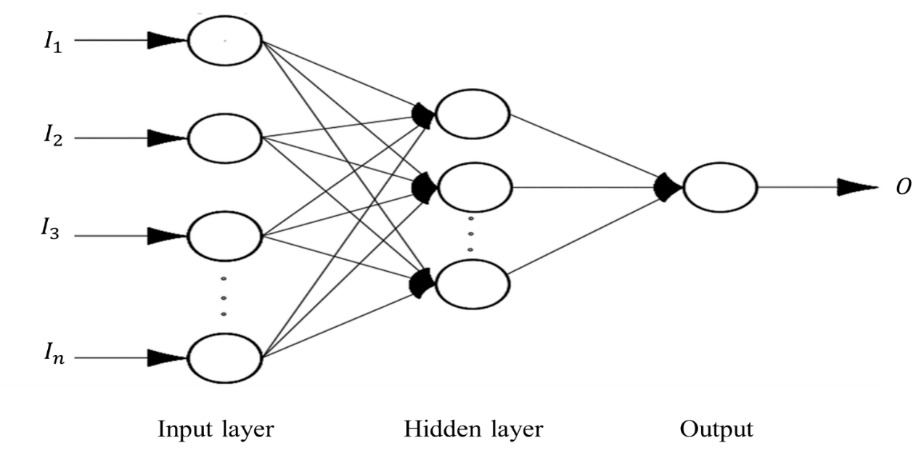Figure 1: Architecture of the Back Propagation Neural Network with an input layer (I), a hidden layer(h) and an output layer(O).

In addition to the above, the hyperbolic tangent activation function used in the hidden layer was employed to introduce a non-linearity into the network. Selection of hyperbolic tangent activation function which produces output in scale of [-1,1]. The hyperbolic tangent activation function used in this study is given in Eq.3 as:(3)

Whereis the sum of the weighted inputs. One of the essential aspects to note about back propagation neural network training is that, it can be characterized as a non-linear optimization problem,as indicated in Eq. (4) as:(4)

whereis the weight matrix andis the error function. To find the optimum weight connectionthat minimizesis the purpose of training the network.

Eq. (2) evaluated at any point ofis given in Eq. (5), Eq. (6), Eq. (7) respectively(5)

whereis the number of training examples andis the output error for each example.is mathematically defined by Eq. (6)(6)

whereandare desired network outputs and estimated values of theoutput neuron for theexample, respectively. Therefore, substituting Eq. (6) into Eq. (5) gives the objective function to be minimized expressed in Eq. (7)  as:(7)

In other to arrive at an acceptable value by the error function, the training process continues by adjusting the weights of the output neurons then proceeds towards the input data. There are several numerical optimization algorithms to perform this weight adaptation . In this study, Levenberg-Marquardt algorithm (LMA) was chosen to train the BPNN because it is faster and has more stable convergence compared to the popular gradient descent algorithm proven in the works of Hagan . The LMA is a gradient descent algorithm. The behavior of the algorithm is like steepest descent method when the current solution is far from the correct one, thus the algorithm approaches the correct solution. Detailed mathematical theory of LMA can be found in [19 and 21].

3.2  Radial Basis Function Neural Network

The RBFNN is a type of ANN which has a feed-forward structure consisting of three layers; the input, hidden and output layers which is indicated in Fig 2. The nodes within each layer are fully connected to the previous layer. Here, the input variables are each assigned to a node in the input layer and transferred to the hidden layer which are unweighted. It can be realized that, the difference between BPNN and RBFNN are that in the RBFNN the connections between the input and hidden layers are unweighted and the activation functions on the hidden layer node are radially symmetric.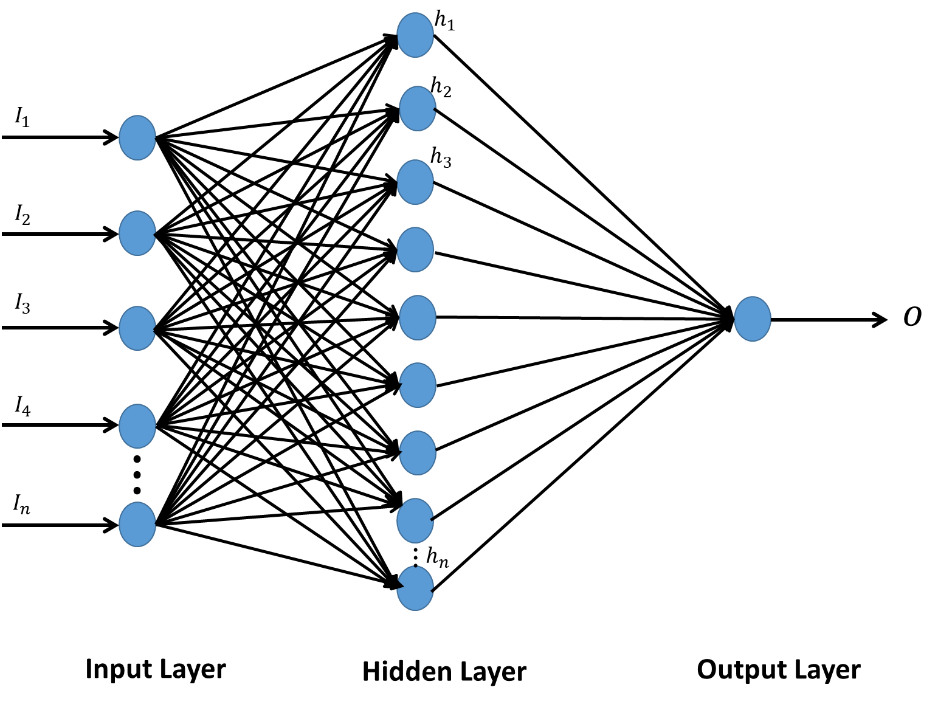Figure 2: Architecture of the RBF Neural Network Based Load Forecasting Technique.

Within the hidden layer, each neuron calculates a Euclidean norm that shows the distance between the inputs. This is then inserted into a radial basis activation function which calculates and outputs the activation of the neuron. In this present study, the Gaussian activation function was employed and it is expressed in Eq. (8) as:(8)

Where,is the input vector,is the center of the Gaussian function andis the spread parameter of the Gaussian bells andis the Euclidean norm. The linear function is contained in the output layer thereby using the weighted sum of the hidden layer as propagation function. Letbe the output of the kth radial basis function on thesample. The output of each target nodeis computed using the weightsindicated in Eq. (9) as:(9)

Here, the target output for sampleon target nodebe. The error functionis expressed in Eq. (10) as:(10)

which has its minimum where the derivative Eq. (11)(11)

vanishes. Let R be the correlation matrix of the radial basis function outputs given by Eq. (12) as follows(12)

The weight matrixEq. 13 which minimizes E lies where the gradient vanishes(13)

Thus, the problem that is solved when the squareMatrix R is inverted, where H represents the number of radial basis function. The singular value decomposition (SVD) approach can be used to solve the matrix inversion whereas diagonalising the matrix provides an approximate inverse. A parameter inverts the eigenvalues which exceeds zero- specified margin and transformed back to the original coordinates, providing an optimal minimum-norm approximation to the inverse in the least-mean-squares sense . This training process continues until the network error reaches an acceptable value.

3.3  Extreme Learning Machine

The ELM model constitutes an input layer, a single-hidden layer, and an output layer. All parameters including the input, weights and hidden bias are determined by iterative network in solving a particular problem [23-24]. As illustrated in Fig 3, the main idea in ELM is that the network hidden layer parameters need not to be learned, but can be randomly assigned. The network output weights can be subsequently and analytically calculated whiles the input weights are randomly chosen. For hidden neurons, there are several activation functions such as sigmoidal, sine, Gaussian and hard-limiting function that can be used, and the output neurons have linear activation function.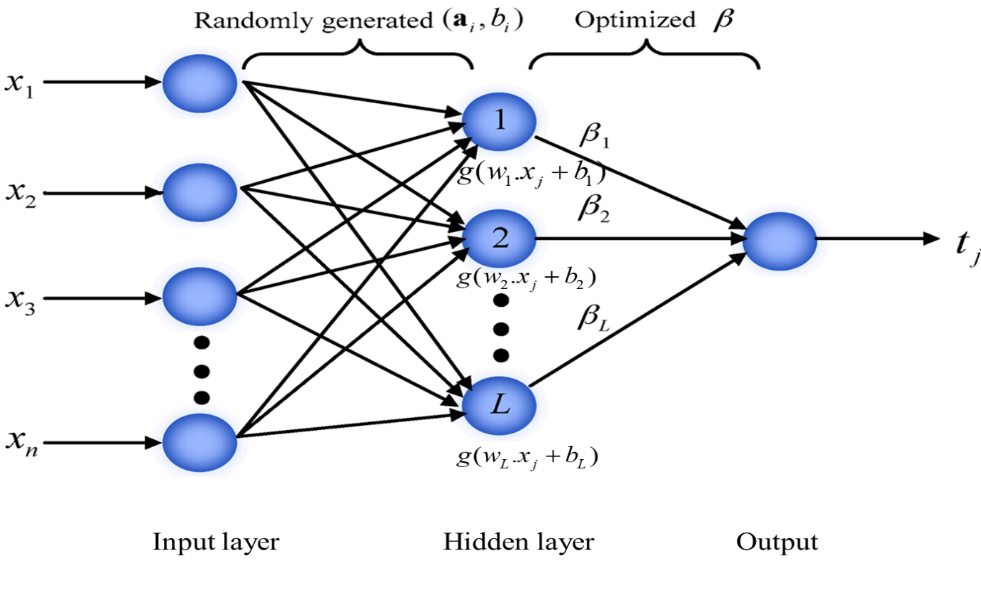Figure 3: Architectural Design of an ELM Predictor.

Briefly, the basic theory of the ELM model states that for N arbitrary distinct input samples (x k, y k) ∈ Rn × Rn, the standard SLFNs with M hidden nodes and an activation function is mathematically described in Eq. (14) as:

(14)where ci ∈ R is the randomly assigned bias of the ith hidden node and wi ∈ R is the randomly assigned input weight vector connecting the ith hidden node and the input nodes. βi is the weight vector connection the ith hidden node to the output node. g (xk; ci, wi) is the output of the ith hidden node with respect to the input sample xk. Each input is randomly assigned to the hidden nodes in ELM network. Then, Eq. (14) can be simplified in Eq. (15) as:(15)

1. ### ANN APPLICATION

4.1  Development of ANN Models

The development of a suitable ANN model to accurately predict the monthly load consumption demand in different sectors is the main aim for this current research. Five sectors: the residential sector, the commercial sector, the industrial sector, the transportation sector and the electric power sector with one balancing item have been taken into consideration. The input and output observed dataset was collected from the U.S. Energy Administration for a period of 44 years from January 1973 through May 2017 and divided into two sub-datasets (training and testing datasets) in a random manner. Yet, extreme measures were taken to prevent repetition of the data in any way.

Three ANN models (BPNN, RBFNN and ELM) for the monthly load consumption prediction were developed after which the performance of the ANN predictions were measured by comparing the prediction outputs with the observed output. The proposed ANN models comprised of three layers being the input layer, the hidden layer and the output layer. Each layer is made up of processing element called neurons. The final output is calculated from the processed neurons in the input and hidden layer.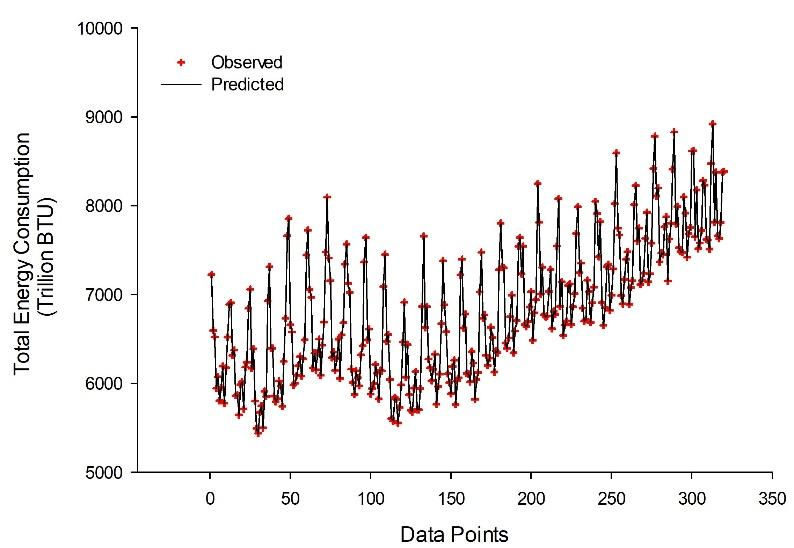Figure 4a: Training data prediction results for backpropagation neural network (BPNN)

To develop the BPNN, RBFNN and ELM models, the dataset for this research was divided into training and testing sets, as explained in section 3. The supervised learning procedure was applied to the three models (BPNN, RBFNN and ELM). With supervised learning, inputs and observed outputs are provided in the models where residential sector, commercial sector, industrial sector, transportation sector, electric power sector as well as the balancing item serves as the input whiles primary energy consumption total serves as the observed output respectively. The data was normalized between [−1 and 1] using a hyperbolic tangent function from the input layer to a single hidden layer for both BPNN. RBFNN model was normalized using the Gaussian function which mimic the non-linearity activation function and an output layer consisting of a linear activation function. Also the ELM model was normalized between [0,1] using the Sigmoid activation function.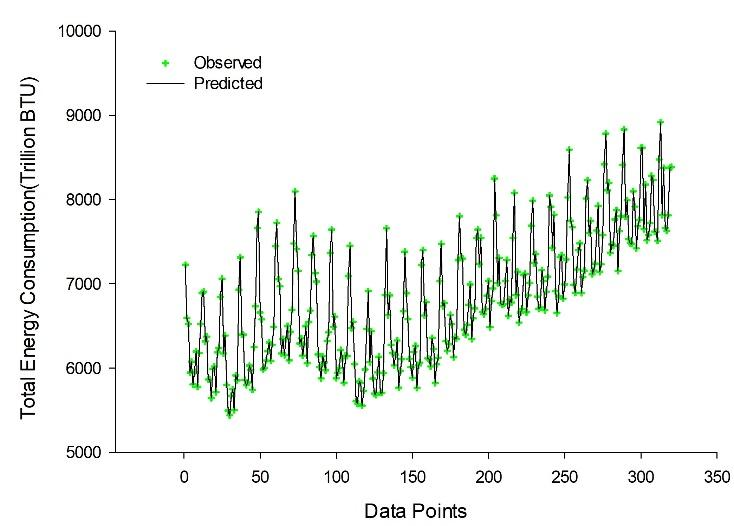Figure 4b: Training data prediction results for radial basis function neural network (RBFNN)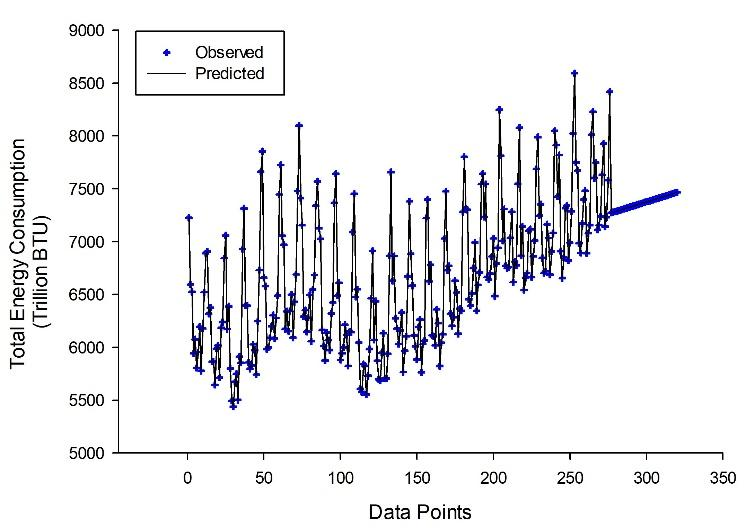Figure 4c: Training data prediction results for extreme learning machine (ELM)

Moreover, after the network was trained for 1000 epochs, using the Levenberg- Marquardt backpropagation algorithm with a learning rate of 0.03 and a momentum coefficient of 0.7 for the BPNN model, the structure constituted of [6-8-1] that is six inputs, one single hidden layer and one output layer. Also, with the RBFNN model, gradient decent learning algorithm was used to train the network through which the weight is adopted in part to the deviation between the observed and the predicted output. The optimum RBFNN structure was [6-40-1, 39] indicating six inputs, forty hidden neurons and one output in addition to 39 spreads. Finally, the ELM model was training using the sigmoid activation function. The optimum ELM structure was [6-461-1] indicating six inputs, four hundred and sixty-one hidden neurons and one output. Fig. 4a indicates the training data prediction results for backpropagation neural network (BPNN) Fig. 4b shows the radial basis function neural network (RBFNN) training data prediction results whiles Fig 4c shows the training data prediction results of the extreme learning machine (ELM). Likewise, the testing data prediction results for backpropagation neural network (BPNN) radial basis function neural network Additionally, for both the training and testing data sets, the structures for BPNN, RBFNN, and ELM models that yielded the best results were chosen as a result of the least mean square error (MSE), the mean absolute error (MAE) and noise to signal ratio (NSR).

4.2. Assessment of Model Performance and Error Statistics

An evaluation was done on the BPNN, RBFNN and ELM models’ performance and error statistics for both training and testing data. This was done by observing the differences between the observed training and testing data that were predicted by BPNN, RBFNN and the ELM models. The performance indicators used in this research were the mean square error (MSE), relative percentage error (RPE) and noise to signal ratio (NSR). In the works of Solmaz & Ozgoren (2012), the mean square error (MSE) and the mean absolute error (MAE) are defined in Eqs. (16) and Eq. (17) and noise to signal ratio (NSR) was added in Eq. (18) as:(16)(17)(18)

whereis the observed monthly load consumption,is the predicted monthly load consumption and N is the total number of data.

The mean square error (MSE) indicates the level of scatter that the ANN model produces. The mean absolute error (MAE) is defined as a quantity used to measure how close the predicted values are to the observed values. And the noise to signal ratio compares the level of the desired signal to the level of background noise.  In other to have a good prediction accuracy of the ANN model, a lower MSE is needed. Nevertheless, the ANN model with lower values of MSE, MAE and NSR is used to evaluate the best model for prediction.

Tables 1 and 2 indicate the BPNN, RBFNN and ELM models’ performance in terms of the mean square error (MSE), mean absolute error (MAE) and noise to signal ratio (NSR) for the observed and predicted load consumption. Training and testing datasets were all evaluated using the MSE, MAE and NSR for the various number of neurons. The best model for predicting the monthly load consumption was selected based on Tables 1 and 2. From the analysis of the results, the optimum model for predicting the monthly load consumption was BPNN in which values obtained in terms of the minimum MAE values were 0.000796433 and 0.008201697 for training and testing results respectively. The minimum value for MSE for BPNN which gave the optimum model for predicting the monthly load consumption were 0.00000143704 and 0.000189 for training and testing results respectively. The minimum value for NSR for BPNN which gave the optimum model for predicting the monthly load consumption were 0.000000175949 and 0.0000016891 for training and testing results respectively. Hence the BPNN proves the best artificial neural network method for predicting the monthly load consumption in this present research. The testing data prediction results for backpropagation neural network, radial basis function neural network as well as extreme learning machine is shown in Fig 5a, 5b and 5c respectively.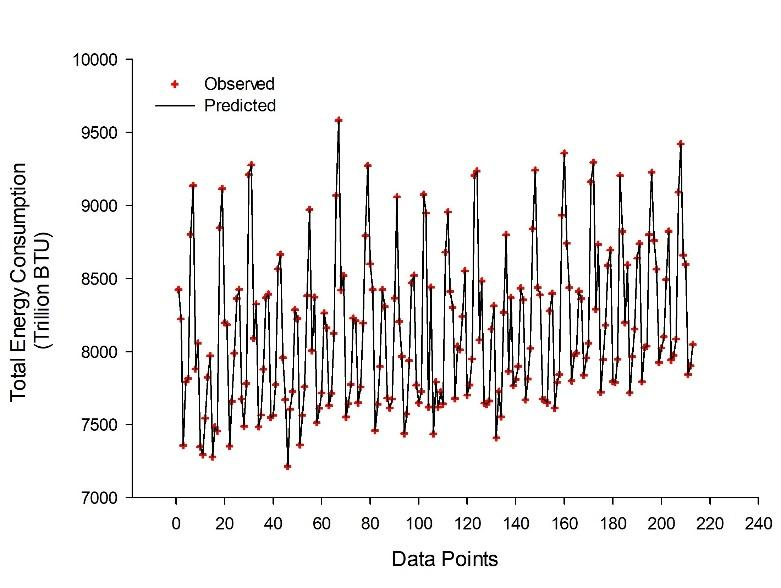Figure 5a: Testing data prediction results for backpropagation neural network (BPNN)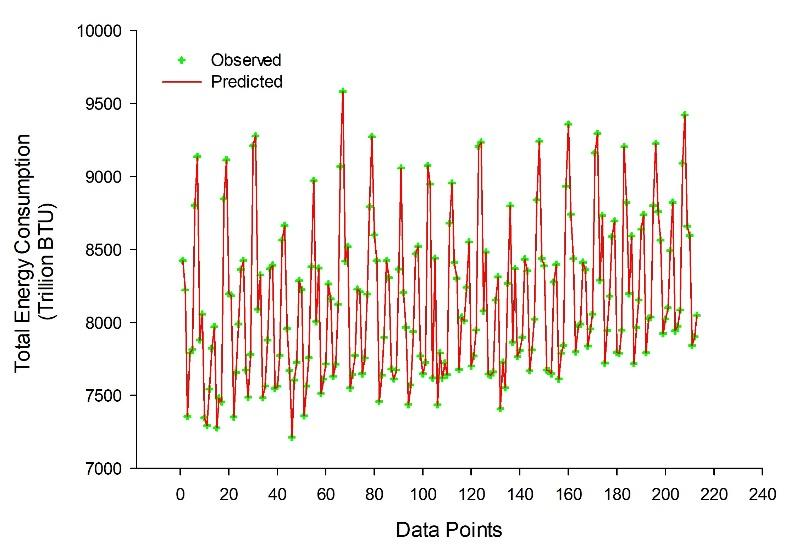Figure 5b: Testing data prediction results for radial basis function neural network (RBFNN)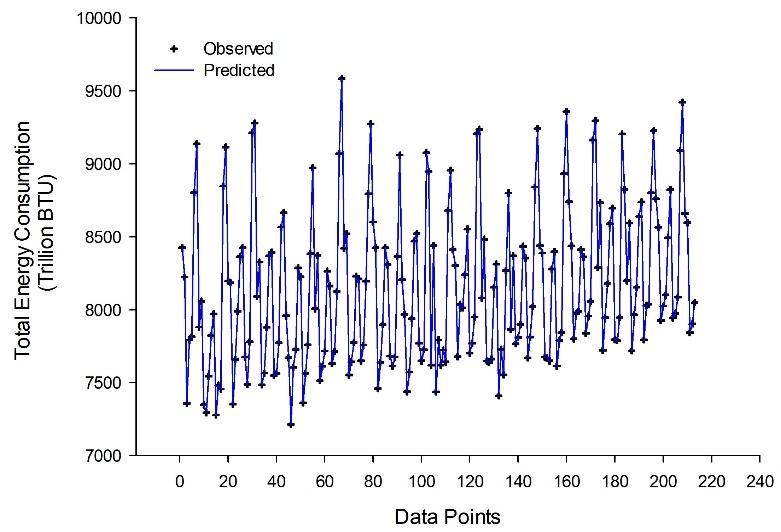Figure 5c: Testing data prediction results for extreme learning machine (ELM)

4.3. Statistics-Based Dimension for Model Efficiency

Likewise, in Table (1-4), three other model efficiency based statistics, relative percentage error (REL), Legates and McCabe index (LM), mean absolute percentage error (MAPE) were also employed to access the model efficiency are expressed in Eqs. (19) to (21) respectively as:(19)(20)(21)

where,is the observed output load consumption,is the predicted output load consumption andis an integer that varies from 1.

Table 1: Model Efﬁciency-Based Statistic Indicators for the Monthly load forecasting for Training

 MODEL BPNN RBFNN ELM MSE 0.00000143704 0.001136 0.001343 MAE 0.000796433 0.025858341 0.028353654 NSR 0.000000175949 0.00000494784 0.00000537913

Table 2: Model Efﬁciency-Based Statistic indicators for the Monthly load forecasting for Testing

 MODEL BPNN RBFNN ELM MSE 0.000189 0.012137 0.824947 MAE 0.008201697 0.102506253 0.729468184 NSR 0.0000016891 0.0000135449 0.000111668

Table 3: Statistical indicators for the Monthly load forecasting (Training Performance)

 MODEL BPNN RBFNN ELM LM 0.999999905 0.999999975 0.000000389 REL 0.999963075 0.998763497 0.998642 MAPE 0.999999885 0.999996136 0.999996

Table 4: Statistical indicators for the Monthly load forecasting (Testing Performance)

 MODEL BPNN RBFNN ELM LM 0.999996092 0.000008314 0.000112 REL 0.999801642 0.997317 0.981328 MAPE 0.999999069 0.999987406 0.999912336

From the analysis of the result shown in Tables 3 and 4, the optimum model for predicting the monthly load consumption was BPNN in which values obtained in terms of the LM values were 0.999999905 and 0.999996092 for training and testing results respectively. The value for REL for BPNN which gave the optimum model for predicting the monthly load consumption were 0.999963075 and 0.999801642 for training and testing results respectively. The value for MAPE for BPNN which gave the optimum model for predicting the monthly load consumption were 0.999999885 and 0.999999069 for training and testing results respectively. Prediction error results training data, testing data for backpropagation neural network (BPNN), radial basis function neural network (RBFNN) and extreme learning machine (ELM) has been shown in Fig. 6a and 6b above.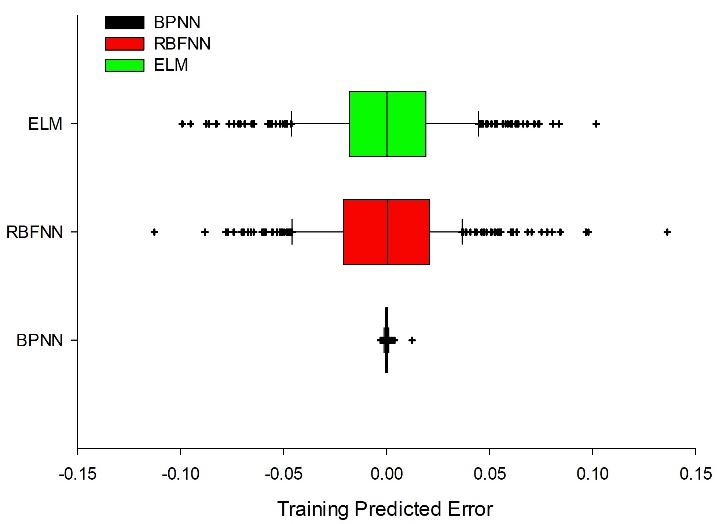Figure 6a: Prediction error results for training data for backpropagation neural network (BPNN), radial basis function neural network (RBFNN) and extreme learning machine (ELM)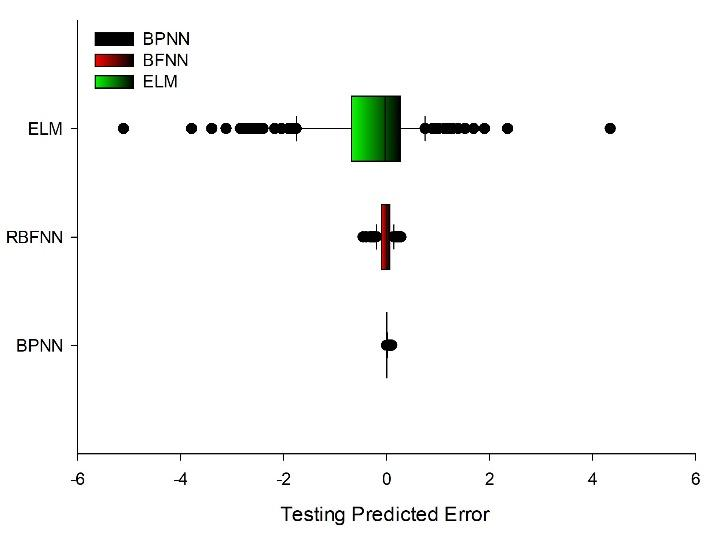Figure 6b: Prediction error results for testing data for backpropagation neural network (BPNN), radial basis function neural network (RBFNN) and extreme learning machine (ELM)

### V.    CONCLUSION

A vital role when considering electrical energy systems, energy supply, planning and operation is an accurate short- term load forecast. This research is aimed to develop an ANN model for accurate prediction of monthly load consumption demand. Therefore, this research has presented the Back Propagation neural network (BPNN), Radial Basis function neural Network (RBFNN) based on supervised learning technique and Extreme Learning Machine (ELM) as a forecasting model to predict a monthly load consumption demand. The dataset was grouped into training and testing data sets. The minimum mean absolute error and MAPE was used as a performance indicator in choosing the optimum models for the load forecasting. Findings from this researched revealed that, the BPNN, RBFNN and ELM offered a satisfactory prediction of the short term monthly load forecasting. Assessment of the models were made by correlating predicted values and observed values where values close to +1 signified a good fit. Also, the mean square errors for both training and testing processes were obtained where errors close to 0 indicated a good prediction. From the analysis of the results, it was found that the predicted values are in good agreement with measured values as the best artificial neural network for predicting the monthly load forecasting is the BPNN comparatively with lower Mean Square Error (MSE) as compared to the RBFNN and the ELM.

This can therefore be concluded that, BPNN is a vital tool to achieve optimum results for short term monthly load forecasting.

### REFERENCES

1. Bhattacharyya, S. C. and Timilsina, G. R. (2009) ‘Energy Demand Models for Policy Formulation a Comparative Study of Energy Demand Models’, Energy, 4866(March), p. 151. doi: 10.1596/1813-9450-4866.
2. Shah, M. and Agrawal, R. (2013) ‘A Review On Classical and Modern Techniques with Decision Making Tools for Load Forecasting’, 6(3), pp. 174–184.
3. Tsekouras, G. J., Kanellos, F. D. and Mastorakis, N. (2015) Short Term Load Forecasting in Electric Power Systems with Artificial Neural Networks. doi: 10.1007/978-3-319-15765-8.
4. Azadeh, A., Seraj, O. and Saberi, M. (2011) ‘An integrated fuzzy regression-analysis of variance algorithm for improvement of electricity consumption estimation in uncertain environments’, International Journal of Advanced Manufacturing Technology, 53(5–8), pp. 645–660. doi: 10.1007/s00170-010-2862-5.
5. Guo, J. J., Wu, J. Y. and Wang, R. Z. (2011) ‘A new approach to energy consumption prediction of domestic heat pump water heater based on grey system theory’, Energy and Buildings, 43(6), pp. 1273–1279. doi: 10.1016/j.enbuild.2011.01.001.
6. Mandal, P. et al. (2006) ‘A neural network based several-hour-ahead electric load forecasting using similar days’ approach’, International Journal of Electrical Power and Energy Systems, 28(6), pp. 367–373. doi: 10.1016/j.ijepes.2005.12.007.
7. Kandil, N. et al. (2006) ‘An efficient approach for short term load forecasting using artificial neural networks’, International Journal of Electrical Power & Energy Systems, 28(8), pp. 525–530. doi: 10.1016/j.ijepes. 2006.02.014.
8. Yao, R. and Steemers, K. (2005) ‘A method of formulating energy load profile for domestic buildings in the UK’, Energy and Buildings, 37(6), pp. 663–671. doi: 10.1016/j.enbuild.2004.09.007
9. Tang, Z. and Fishwick, P. a. (1993) ‘Feedforward Neural Nets as Models for Time Series Forecasting’, INFORMS Journal on Computing, 5(4), pp. 374–385. doi: 10.1287/ijoc.5.4.374.
10. US Energy Information Administration (2016) How much electricity is used for lighting in the United States? - FAQ - U.S. Energy Information Administration (EIA), www.eia.gov. Available at: https://www.eia. gov/tools/faqs/faq.cfm?id=99&t=3.
11. Kohzadi, N. et al. (1996) ‘A comparison of artificial neural network and time series models for forecasting commodity prices’, Neurocomputing, 10(2), pp. 169–181. doi: 10.1016/0925-2312(95)00020-8.
12. Hagan, M. T. and Menhaj, M. B. (1994) ‘Training Feedforward Networks with the Marquardt Algorithm’, IEEE Transactions on Neural Networks, 5(6), pp. 989–993. doi: 10.1109/72.329697.
13. Azadeh, A., Ghaderi, S. F. and Sohrabkhani, S. (2007) ‘Forecasting electrical consumption by integration of Neural Network, time series and ANOVA’, Applied Mathematics and Computation, 186(2), pp. 1753–1761. doi: 10.1016/j.amc.2006.08.094.
14. Worrell, E., Ramesohl, S. and Boyd, G. (2004) ‘Advances In Energy Forecasting Models Based On Engineering Economics’, Annual Review of Environment and Resources, 29(1), pp. 345–381. Doi:10. 1146/annurev.energy. 29.062403.102042.
15. Karl, T. R. et al. (2010) ‘Observation needs for climate information, prediction and application: Capabilities of existing and future observing systems’, in Procedia Environmental Sciences, pp. 192–205. doi: 10.1016/j.proenv.2010.09.013.
16. Larochelle, H. et al. (2009) ‘Exploring Strategies for Training Deep Neural Networks’, Journal of Machine Learning Research, 1, pp. 1–40. doi: Doi 10.1109/Tsmcc.2012.2220963.
17. Hornik, K., Stinchcombe, M. and White, H. (1989) ‘Multilayer feedforward networks are universal approximators’, Neural Networks, 2(5), pp. 359–366. doi: 10.1016/0893- 6080(89)90020-8.
18. Konaté, A. A. et al. (2014) ‘Prediction of porosity in crystalline rocks using artificial neural networks: An example from the Chinese Continental Scientific Drilling Main hole’, Studia Geophysica et Geodaetica, 59(1), pp. 113–136. doi: 10.1007/s11200- 013-0993-5.
19. Milanič, M. et al. (2011) Numerical optimization of sequential cryogen spray cooling and laser irradiation for improved therapy of port wine stain, Lasers in Surgery and Medicine. doi: 10.1002/lsm.21040.
20. Hagan, M. T. and Menhaj, M. B. (1994) ‘Training Feedforward Networks with the Marquardt Algorithm’, IEEE Transactions on Neural Networks, 5(6), pp. 989–993. doi: 10.1109/72.329697.
21. Lourakis, M. I. a (2005) ‘A Brief Description of the Levenberg-Marquardt Algorithm Imple mened by levmar’, Matrix, 3, p. 2. doi: 10.1016/j.ijinfomgt.2009.10.001.
22. Michie D, Spiegelhalter DJ, Taylor CC (1994) Machine learning, neural and statistical classiﬁcation. Ellis Horwood, Upper Saddle River, NJ, USA.
23. Parikh, P. J. and Lam, S. S. (2009) ‘Solving the forward kinematics problem in parallel manipulators using an iterative artificial neural network strategy’, International Journal of Advanced Manufacturing Technology, 40(5–6), pp. 595–606. doi: 10.1007/s00170-007-1360-x.
24. Huang, G., Zhu, Q. and Siew, C. (2004) ‘Extreme Learning Machine: A New Learning Scheme of Feedforward Neural Networks’, IEEE International Joint Conference on Neural Networks, 2, pp. 985–990. doi: 10.1109/IJCNN.2004.1380068.#### For Authors

/symposiums/webinars, networking opportunities, and privileged benefits.
Authors may submit research manuscript or paper without being an existing member of LJP. Once a non-member author submits a research paper he/she becomes a part of "Provisional Author Membership".

Know more#### For Institutions

Society flourish when two institutions come together." Organizations, research institutes, and universities can join LJP Subscription membership or privileged "Fellow Membership" membership facilitating researchers to publish their work with us, become peer reviewers and join us on Advisory Board.

Know more#### For Subscribers

Subscribe to distinguished STM (scientific, technical, and medical) publisher. Subscription membership is available for individuals universities and institutions (print & online). Subscribers can access journals from our libraries, published in different formats like Printed Hardcopy, Interactive PDFs, EPUBs, eBooks, indexable documents and the author managed dynamic live web page articles, LaTeX, PDFs etc.

Know more